×

Search anything:

# Understanding Fenwick tree (Binary Indexed Tree) with Range product

#### Data Structures tree data structure fenwick treeGet this book -> Problems on Array: For Interviews and Competitive Programming

Reading time: 40 minutes | Coding time: 10 minutes

Fenwick tree is a tree based data structure that is widely used to solve range query problems in logarithmic time O(log N) which would otherwise have taken linear time O(N). In this article, we have explained it in depth using the range product query problem.

• Fenwick tree was developed by Peter Fenwick in 1994 to improve the efficiency of arithmetic coding compression algorithms.
• Any number can be represent as sum of power of 2.This is the basic concept behind Fenwick tree.
• Each node contain index and value.
• We can compute prefix product(product of first i elements 0<=i<n) of an array using Fenwick tree.this prefix product is useful to compute range product of an array.

We explained Fenwick Tree using the Range product problem. In this article first we will discuss:

1. How does Fenwick Tree work ?
2. How to construct Fenwick tree?
3. How to find prefix product ?
4. How to find range product using prefix product ?
5. Coding Implementation
6. Applications of Fenwick tree.

## How does Fenwick Tree work?

• Any number can be represent as sum of power of 2.This is the basic concept behind Fenwick tree.
• Each element whose index i is a power of 2 contains the product of the first i elements.
• Elements whose index are the sum of two (distinct) powers of 2 contain the product of the elements since the preceding power of 2.
• Example :
• Note : [x,y) it means (elements between x(include) and y(exclude)).
1. index i=1
1=0+20=0+1
It means index 1 contains product of [0,1).
2. index i=2
2=0+21=0+2
It means index 2 contains product of [0,2).
3. index i=7,
7=22+21+20=6+1
It means index 7 contains product of [6,7).

## Construction of Fenwick tree

• root of tree is dummy.
• we can represent Fenwick tree as an array. we start inserting elements from 1st index.
• each node of the tree has an index and value.
• To construct Fenwick tree first we initialize all node with 1.
• FT[] is an array of Fenwick tree. we represent node's index in terms of sum of power of 2.
• now based on this representation we store the value into that node. for example index i=2 can represent as 0+2 so we store product of index [0,2).

## Pseudo code to Construct Fenwick tree :

``````function update(value,i,n,oldvalue)
i=i+1
while i&lt=n
FT[i]/=oldvalue
FT[i]*=value
i=i+(i&(-i))
endwhile
endfunction
function construct(arr[],n)
declare FT :ARRAY[0,n] of INT
declare i :INTEGER
i = 0
for i=0 to FT.length
FT[i]=1
end
for i=0 to FT.length
update(FT[i],i,n,1)
end
endfunction
``````
• Example :

• Input array a={1,2,3,4,5,6,7}.

• i=0

• Initialize all node value=1

• go to update function

1. i=i+1=1
2. 1<=7
3. FT=1
4. i=2 {(1+(1&(-1)))=2}
• repeat the above process from step 2 till i <= n.

•• i=1

•• i=2

•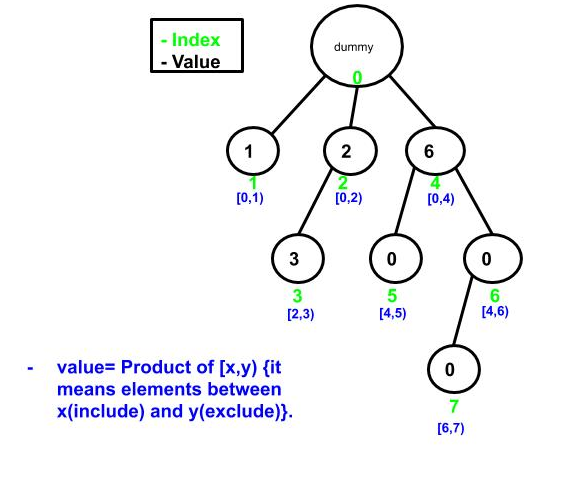• when we reached at i=6 all node of the tree is filled up with it's
value.

• FT={0,1,2,3,24,5,30,7}.

•## Steps to find prefix product

1. to find product of first i elements of an array we need value and index of parent node.
• steps to find parent node :
1. take 2's complement of i (i is index of that node).
2. perform and operation between i and 2's complement of i.
3. subtract the result of step 2 from i.
4. parent node= i-(i&(-i)).
• Example :
array=[1,2,3,4,5,6,7].
• process to compute parent of 1(index i=1) :
1. 2 in binary(8 bit representation) =00000001
2's complement of 1 =11111111
2. 11111111 & 00000001=00000001.
3. 00000001 - 00000001=0.
• process to compute parent of 3(index i=3) :
1. 3 in binary(8 bit representation) =00000011
2's complement of 3 =11111101.
2. 00000011 & 11111101=00000001.
3. 00000011 - 00000001=00000010=2(decimal).
• short trick to find parent node :
• parent of any node can be obtain by removing the last set
bit from the binary representation of that node.
• which is nothing but i-(i&(-i)).
• Example :
• 5 in binary 101.simply remove last set bi it will
become 100=4. 4 is parent of that node.
• Pseudo code to find parent node :
``````
function parentnode(i)
i = i-(i&(-i))
return i
endfunction
``````
1. go to i th node multiply it's value with product(product is variable) .
2. traverse to it's all ancestors node. multiply each ancestors node's value with product.
• example :
• Input array a={1,2,3,4,5,6,7}.
• let's say we want to find product of first 7 elements .
• Fenwick tree for given input array.
•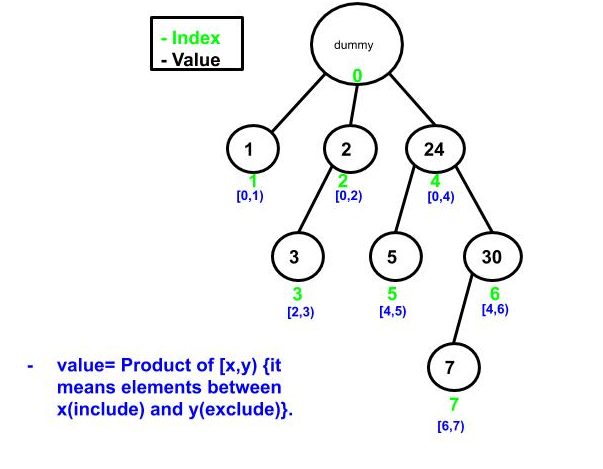• product is variable whose initial value is 1.
• go to node 7(7 is node index) multiply node value with product.
• anscestors of node 7 is 6 and 4.
• move to it's ancestor node which is 6(6 is parent node of 7) multiply node value with product.
• now parent of 6 is 4 multiply value with product.
• parent of 4 is dummy so stop it.
•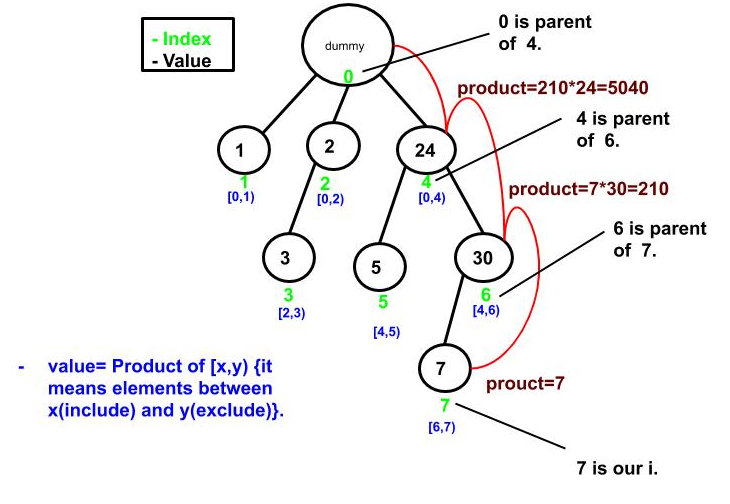## Pseudo code to find prefix product

``````function product(i)
declare product : INTEGER
product =1
i=i+1
while i is greater than 0
product*=FT[i]    //FT is an array for fenwick tree
i = parentnode(i) //(parentnode(i) returns parent of i)
endwhile
endfunction
``````

## Range product :

• Range product of an array means product of given range.
• We could find Range product using prefix product(product of first i elements).
• Range product query have an higher and lower index(l,h).
• l and h is index of an input array, so when we pass it to product function first it will increment by 1, because in FT[](fenwickktree array) we stores element from index 1.
• prefix product of any higher index=array×array×...×array[l]×...×array[h].
• for range product we just need array[l]×array[l+1]× ...×array[h].
• So we need to remove unnecessary elements from prefix product of higher index.
• this unnecessary element is nothing but prefix product of lowerindex-1.
• compute prefix product of lowerindex-1, then divide prefix product of higher index with prefix product of lowerindex-1.
• hence range product = $\frac{\mathrm{\left(array\left[0\right]×array\left[1\right]×...×array\left[l\right]×...×array\left[h\right]\right)}}{\mathrm{\left(array\left[0\right]×array\left[1\right]×...×array\left[l-1\right]\right)}}$
• Range product(l,h)=$\frac{\mathrm{product\left(h\right)}}{\mathrm{product\left(l-1\right)}}$

### Steps to find Range Product :

``````1. Compute Prefix product of higher index using product function (product(h)).
2. Compute Prefix product of lowerindex-1 using product function (product(l-1)).
3. divide prefix product of higher index(product(h)) with prefix product of lowerindex-1(product(l-1)).
``````
• Example :
• Input array a =[1,2,3,4,5,6,7].
• let's say we want to find range product of a[3,5].
• Fenwick tree of an input array
•• Range product(l,h)=$\frac{\mathrm{product\left(h\right)}}{\mathrm{product\left(l-1\right)}}$
• prefix product of higher index (product(5)) =720
•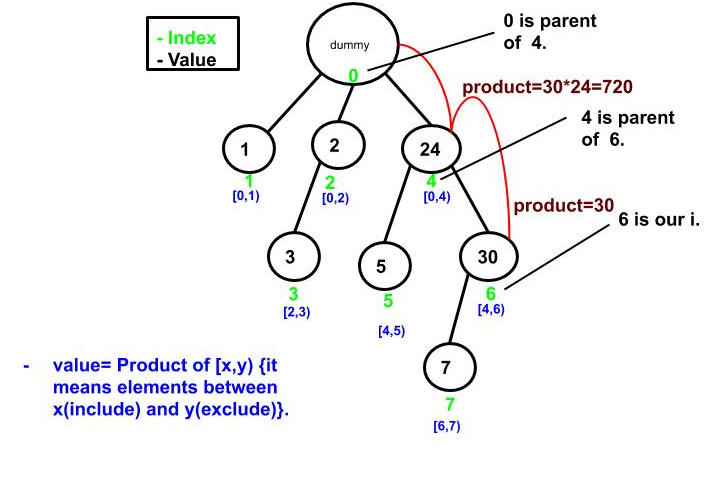• prefix product of (lower index-1)(product(2)) =6
•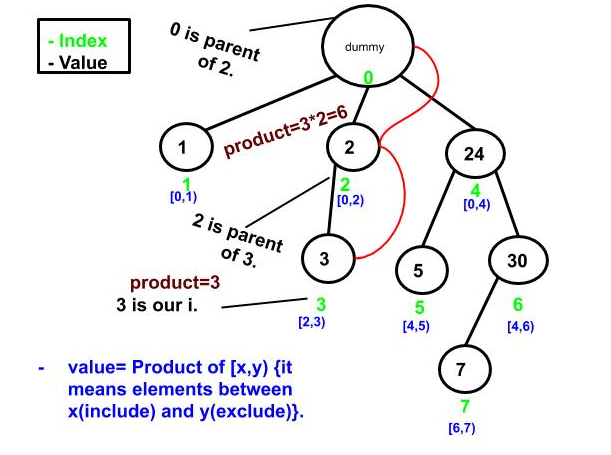• hence range product of a[4,6]=7206=120

## implementation :

``````import java.util.Arrays;
public class FenwickProduct {
public static int n=0;
public static int FT[];
public static void main(String[] args) {
int a[] = {1,2,3,4,5,6,7};
n=a.length;
FT =new int[n+1];  //create an arrray for fenwick tree
construct(a);  //construct fenwick tree
System.out.println("Fenwick array");
System.out.println(Arrays.toString(FT));
System.out.println("product of First seven elements of an array [0,...6] is ="+product(6));
System.out.println("Range product of [3,5] is ="+rangeproduct(3,5));
}
public static void construct(int arr[])  // create a Fenwick tree of given array
{
for(int i=1; i&lt=n; i++)  // initialize all node with one
{
FT[i]=1;
}
// Store the values in FT[] using update()
for(int i=0; i&ltn; i++)
update(i,arr[i],1);
}
public static void update(int i,int value,int old)  // multiply value to element with index i
{
i=i+1;
while(i&lt=n)
{
FT[i]/=old;
FT[i]*=value;
i=i+(i&(-i));
}
}

public static int product(int i)  //  returns the product of input array[0,..i]
{
int product=1;
i=i+1;
while (i&gt0)
{
product*=FT[i];
i=parentnode(i);
}
return product;

}
public static int rangeproduct(int p,int q) // q>p return the range product
{
int rangeproduct=(product(q)/product(p-1));
return rangeproduct;
}
public static int parentnode(int i) // returns the parent node(index) of index i
{
int index = i-(i&(-i));
return index;
}
}
``````

### Output :

``````Fenwick array
[1, 1, 2, 3, 24, 5, 30, 7]
product of First seven elements of an array [0,...6] is =5040
Range product of [3,5] is =120
``````

## Key Point :

• Fenwick tree construction time complexity O(nlogn).
• product and update will take at most O(logn) time.
• Space complexity is O(n).
• range product can be computed in O(logn).

## Applications of Fenwick tree

• Fenwick tree are used to implement the arithmetic coding compression algorithm.
• Fenwick Tree can be used to count inversions in an array in O(nlogn) time.
• Sum of range can be computed using Fenwick tree.#### Akshay Gopani

Intern at OpenGenus (2019) | Bachelor of Technology (2017 to 2021) in Information and Communication Technology at Ahmedabad University